#### Risk Disclosure

Futures, foreign currency and options trading contains substantial risk and is not for every investor. An investor could potentially lose all or more than the initial investment. Risk capital is money that can be lost without jeopardizing ones financial security or lifestyle. Only risk capital should be used for trading and only those with sufficient risk capital should consider trading. Past performance is not necessarily indicative of future results. View full risk disclosure.

## Threshold Solver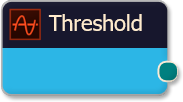The Threshold solver compares the Input value against any number of threshold levels.  This solver is primarily used to identify when an indicator is above or below a certain value (a threshold value).  e.g.  This solver would be used to identify the following conditions; when the CCI is above 100 or below -100.  Or, when the Stochastics is above 80 or below 20, and vise-versa.  Or, if the MACD is above zero or below zero.  Those numeric values are threshold levels/values.
Tip:  If you want to compare an indicator to another indicator, or bar prices, or some other non-fixed numeric value, then use the Comparison solver.

# BloodHound   v2

This section is specific to BloodHound v2.  Scroll down for BloodHound v1.

## Properties tab

This tab contains the essential settings for all nodes.

### Modifiers

Please note:   The buttons only modify the individual instance of the selected node(s) on a Logic Board.   Thus, the buttons are only visible when a node is selected on the Logic Board.  The buttons are not available when a solver is selected in the Solvers panel, because the original solver's output can not be globally modified.
This behavior is consistent with adding a function node afterwards, so that the original solver's output remains unmodified elsewhere in the system.  It is similar to having an SMA(50) on several charts.  Changing the plot color on one chart does not modify the plot color on the other charts.

### Global Properties

These setting apply to all instances of the selected solver.  Changing a setting in one of the instances changes the setting in all instances.  If you only want to change one of the solvers, but not the others, you must make a copy of the solver.  The copy will be a different solver, and a number will be added at the end of the name to differentiate it from the original.

### Behavior

This option determines which outputs are calculated.  Effectively turning off either the Long or Short output.

#### Input

This section determines what kind of data the solver will evaluate.  Use the Type drop-down menu to select the data type.
The menu will change based on which data type is selected. Click on Type to see the various data types available and the corresponding documentation.

The Input section determines the data type to be evaluated.  Use the 'Type' menu to select the data type.  See below for detailed information on each data type.

##### Type:   Price

This option allows price data to be used in the solver.  The price data can be shaped/manipulated by the various settings before it is used by the solver.

Custom Prices
SharkIndicators has custom system development prices that are very useful in reducing the number of solvers, and simplifying system logic, when evaluating the open and close (body) prices.
Body Top:  Regardless of the bar direction, this returns the upper price of the candlestick body.  Either the close or open price.  Whichever is on top.  e.g.  For an up bar, the Body Top equals the close price.
Body Median:    This returns the median price of the candlestick body.  The formula is (Close + Open) ÷ 2.
Body Bottom:    Regardless of the bar direction, this returns the lower price of the candlestick body.  Either the close or open price.  Whichever is the bottom.  e.g.  For an up bar, the Body Bottom equals the open price.

##### Type:   Volume

Volume will feed the current bar volume into the solver.
To evaluate the volume from a few bars back, use Indicator Value.  Then select the VOL indicator, and use the Displacement setting.

##### Type:   Swing Point Prices

Swing Point Prices uses the Swing Highs & Lows indicator plots for input price data.  This is useful when you need to access the lowest low or highest high prices from the latest swing point.
You can add the bundled indicator Swing Highs & Lows to the chart to help you visualize the price data the solver is using.

## Options tab

This tab contains extra secondary settings common to all nodes.

### Weight

This section is used to modify the node's final output value.  It is only useful for designing fuzzy logic systems, or a fuzzy logic section of a system.
Please note:   The Weight controls only modify the individual instance of the selected node(s) on a Logic Board.   Thus, the Weight controls are only visible when a node is selected on the Logic Board.  The controls are not available when a solver is selected in the Solvers panel, because the original solver's output can not be globally modified.
This behavior is different than BloodHound 1.0.  In BH 1.0, the Weight controls modified the nodes output globally (every instance).  This change in BH 2.0 adds more system design granularity.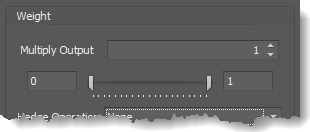Multiply Output:   This takes the internal values (the Long and Short values determined via the Properties tab » Output Rules section) and multiplies them by this value.  Note: the final output will not exceed a value of 1, as described in the Slider control below.

Slider control:  The slider constrains the final output to a value of 0 to 1.  The left side of the slider sets a minimum value that is output regardless if the solver condition is true or not.  The right side sets a maximum value that is output.  The output is capped.
e.g.  Three indicator conditions are being checked, and thus three solvers are created.  Only two out of the three indicator conditions are needed.  An Additive logic node is used to add the solver's outputs together.  Just two out of the three solvers need to add up to a value of 1.  Therefore, the right slider (max output value) for all three solvers is set to 0.5.  When two indicator/solver conditions are found, thus the outputs = 0.5, then the calculation, in the Additive node, is 0.5 + 0.5 + 0 = 1.  A value of 1 means the two out of three condition is true.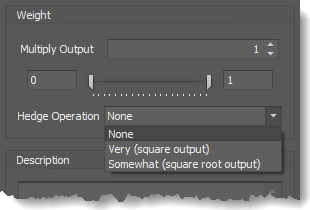Hedge Operation: This applies a mathematical formula to the internal value.

None:  No modification is applied.
Very (square output):  A squaring formula is applied.  Output  =  value .
Somewhat (square root output):  A square root formula is applied.  Output  =  √value .

### Description

This text area provides a place to write a full description of what the node is doing, used for, or what ever you want.
Note:   The Description is global to all instances of the node.  It is not applied individually to each instance as the Weight controls are.

## Help tab

This tab displays the documentation page (from this web site) of the selected node.
Please note:  NinjaTrader v8.0.26.0 or newer is required for the built in web viewer to work, and thus the documentation to be displayed.

### Examples

#### Example 1A: The Basics

This demonstrates a basic function of the Threshold solver using the Stochastics » D plot.    This Solver detects overbought, above 80, and oversold, below 20, conditions of the Stochastics » D.

• Set Indicator to Stochastics » D.

Rules window Settings:
Open the Rules window by clicking the ellipses button in section Output » Threshold Settings.

• Set Thresholds values as shown.

The chart below shows a Long output when the Stochastics D is above 80, and a Short output when it is below 20.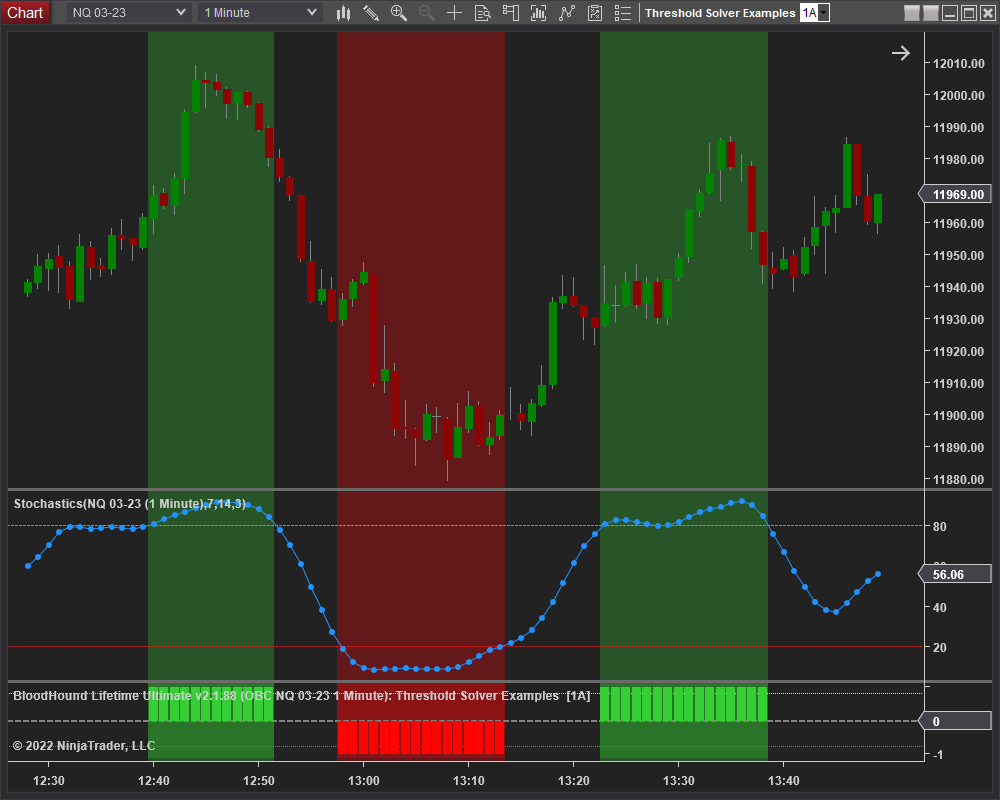#### Example 1B: The Basics with Volatility

This demonstrates a basic function of the Threshold solver to detect volatility.    This Solver detects when the ATR is above 7.  Some systems may need or take advantage of increased volatility to work well.  The chart below shows when an increase in active trading picks up.

• Set Indicator to ATR.

Rules window Settings:
Open the Rules window by clicking the ellipses button in section Output » Threshold Settings.

• Set Thresholds values as shown.
• Note the Long & Short settings are the same.

The chart below shows a Long & Short output when the ATR goes above 7.  The ATR is a non-trend directional indicator, which is why there is a Long & Short output at the same time.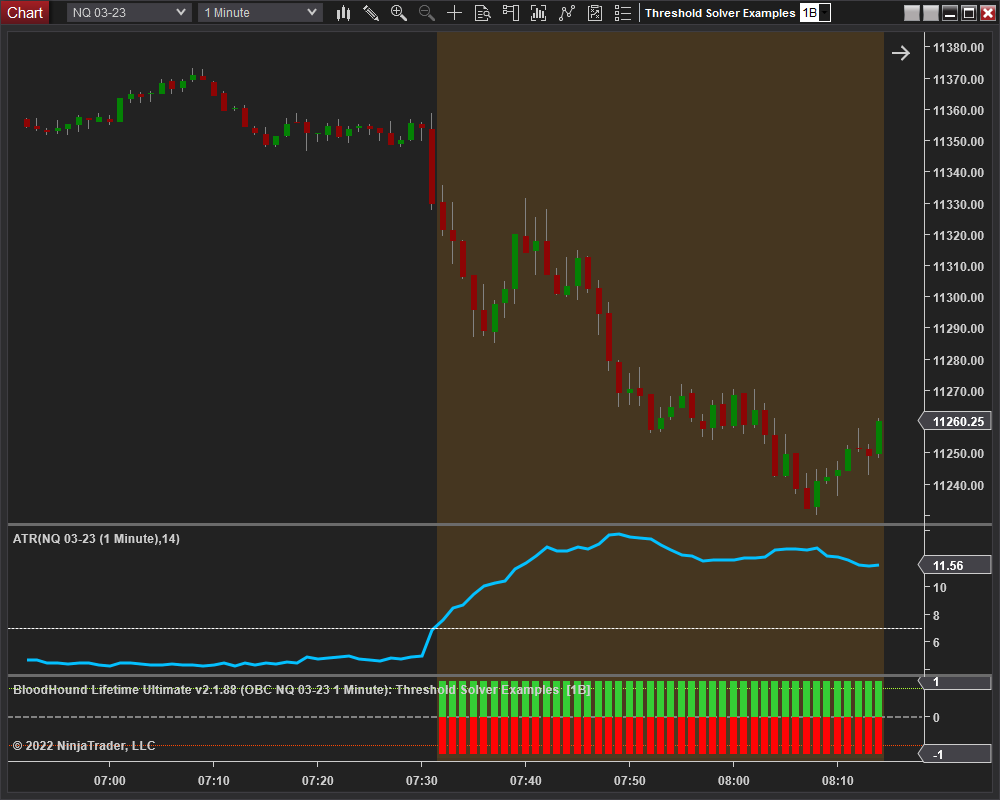#### Example 1C: A Basic MACD Filter

This demonstrates another basic function of the Threshold solver using the MACD line.  This Solver will identify if the MACD is above the zero line for a long filter, or below the zero line for a short filter.  The zero line threshold of the MACD switches the output between Long and Short.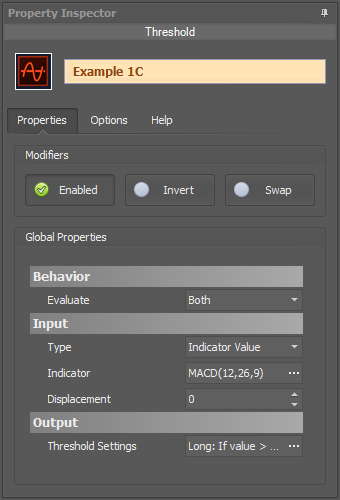• Set Indicator to the MACD.

Rules window Settings:
No changes to the Rules window is necessary.

The chart below shows a Long output when the MACD is above zero, and a Short output when it is below zero.  Quite a simple filter.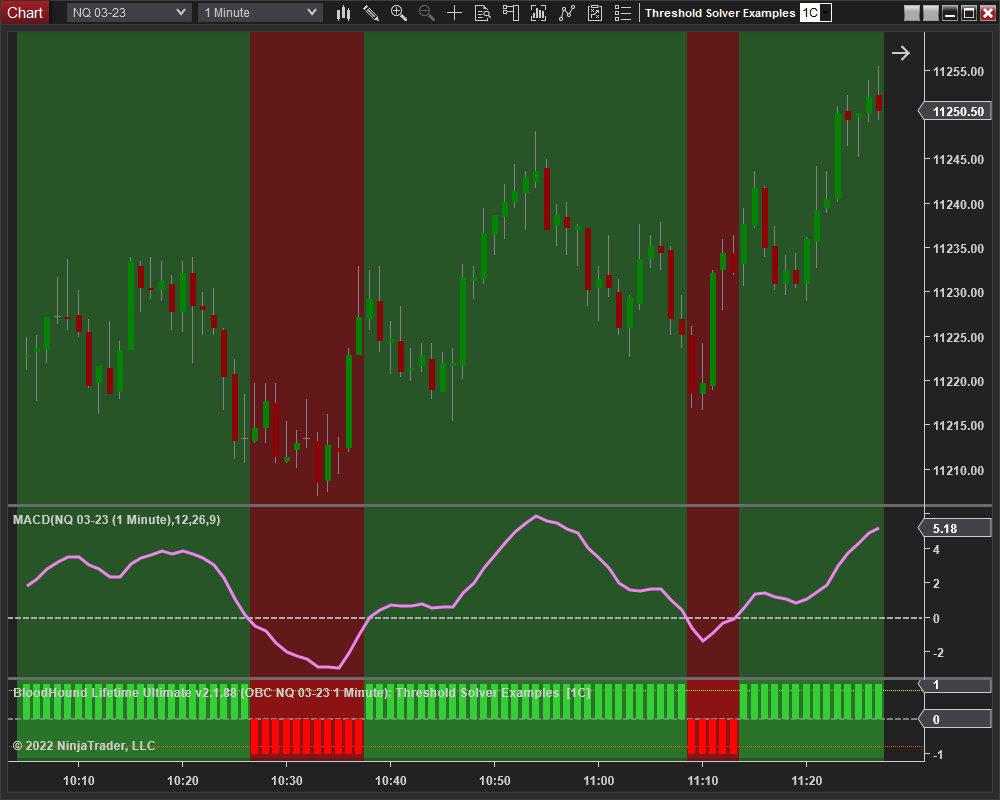#### Example 2A: Using A Discretionary S/R Level

This demonstrates a simple way to use a discretionary support/resistance level to filter long only trading above a price level, or shorts only below that price level.  The Solver identifies when the closing price is above or below the discretionary level.

• Set Input » Type to Price.

Rules window Settings:
Open the Rules window by clicking the ellipses button in section Output » Threshold Settings.

• Set Thresholds values as shown.

The chart below shows a Long output when the close price is above 11,200.0, or a Short output when the close is below 11,200.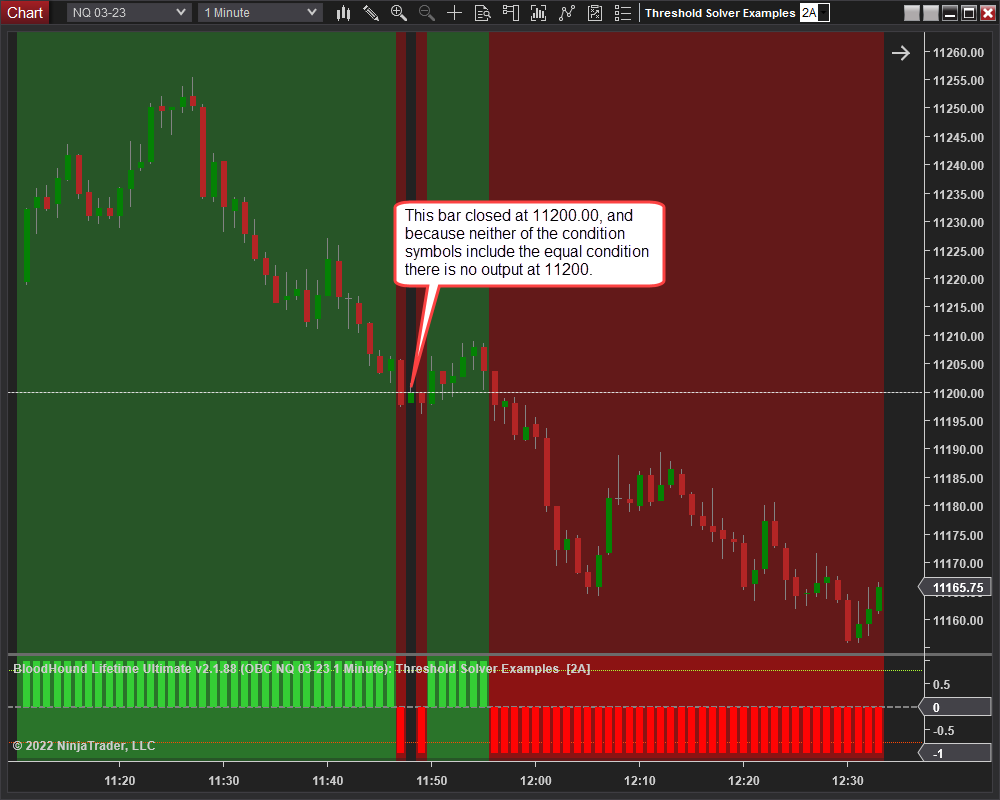#### Example 2B: Two Discretionary S/R Levels

This demonstrates using two discretionary support/resistance levels.  When the market (closing bar price) is above the lower S/R level a long only filter is applied. When the market is below the upper S/R level a short only filter is applied. When the market is between the two levels both long and short signals can occur.

• Set Indicator » Type to Price.

Rules window Settings:
Open the Rules window by clicking the ellipses button in section Output » Threshold Settings.

• Set Thresholds values as shown.

The chart below shows a Long output when the close price is above 11,160.0, a Short output when the close is below 11,200, and of course a Long & Short output when the close is between the two levels.#### Risk Disclosure

Futures, foreign currency and options trading contains substantial risk and is not for every investor. An investor could potentially lose all or more than the initial investment. Risk capital is money that can be lost without jeopardizing ones financial security or lifestyle. Only risk capital should be used for trading and only those with sufficient risk capital should consider trading. Past performance is not necessarily indicative of future results. View full risk disclosure.

# BloodHound   v1

This section is specific to BloodHound v1.  Scroll up for BloodHound v2.

# Indicator Threshold SolverThe Threshold solver compares the Input value against 5 possible threshold levels.  This solver is primarily used to identify when an indicator is above or below a certain value (a threshold value).  e.g.  This solver would be used to identify the following conditions; when the CCI is above 100 or below -00.  Or, when the Stochastics is above 80 or below 20.  Or, if the MACD is above zero or below zero.  Those numeric values are threshold levels/values.
When the input value falls between threshold levels the output value is calculated by linearly interpolating between the corresponding Output values.
Tip:  If you want to compare an indicator to another indicator, or bar prices, or some other non-fixed numeric value, then use the Comparison solver.

### Parameters

#### Thresholds

Please note, Threshold values must be entered in descending order.
I.E  #1  A = 5, B = 4, C = 3, D = 2, E = 1
#2  A = 2, B = 1, C = 0, D =  -1, E =  -2

#### Short Output

Sets the solver’s Short output values as described in Long Output above.

### Video Tutorial

This video is from our weekly Workshop on July 10th, 2015.
For more benefit please watch in full screen mode, as this video is recorded in HD.

### Examples

#### Example 1A: The Basics

This demonstrates the basic function of Indicator Threshold solver using the Stochastic D.    This Solver will be used to detect over-bought, above 80, and over-sold, below 20, conditions of the Stochastic D.    In this first example the 50 line of the Stochastic will be used to switch the output between Long and Short.• Add the Indicator Threshold solver
• Set Indicator to Stochastic D
• Set Thresholds values as shown
• Set Long Output > Greater Than 80 to 1
• Set Short Output > Less Than 20 to 1

The chart below shows a Long output when the Stochastic D is above the 50 line, and a Short output when it is below the 50 line.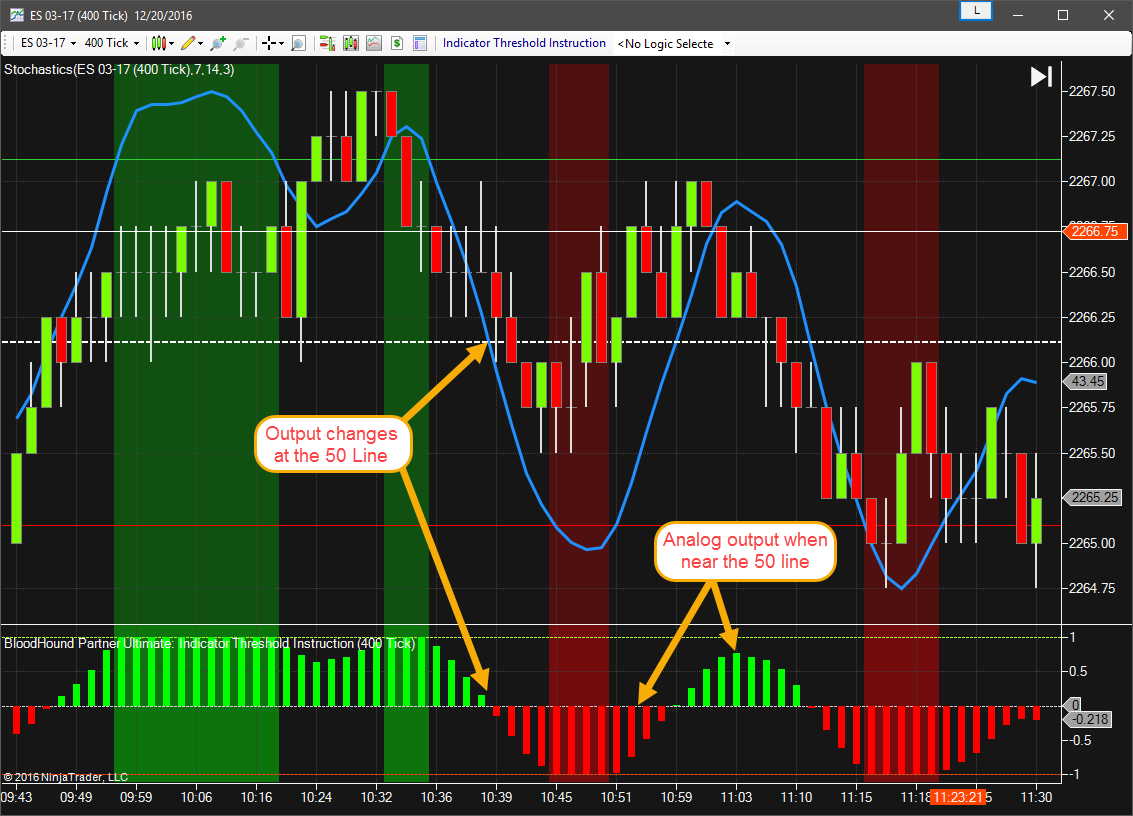#### Example 1B: The Basics of Going Digital

This demonstrates the basic function of Indicator Threshold solver using the Stochastic D.    This time the Solver will be set to give a digital style output.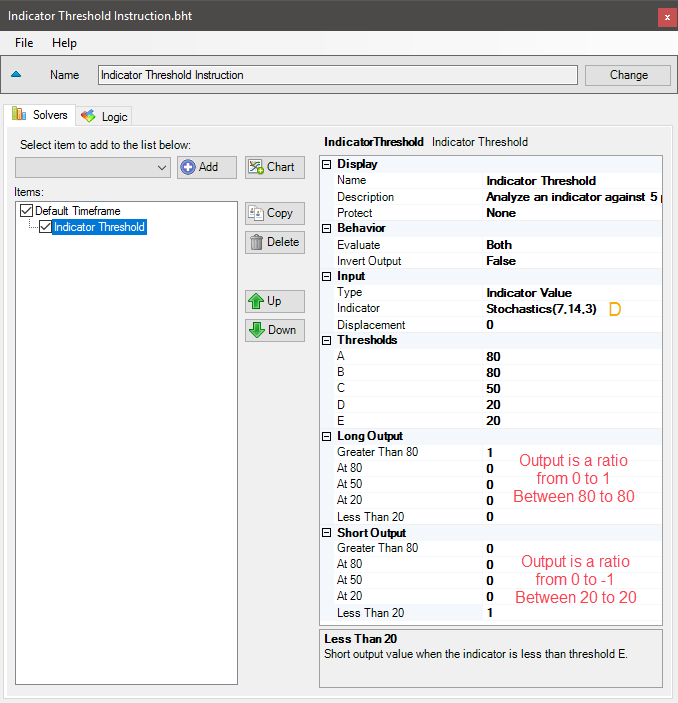• Add the Indicator Threshold solver
• Set Indicator to Stochastic D
• Set Thresholds values as shown
• Set Long Output > Greater Than 80 to 1
• Set Short Output > Less Than 20 to 1

Notice that At B & At C are set to 0, and thus no output values between 20 to 80.    The chart below only shows values above 80 and below 20.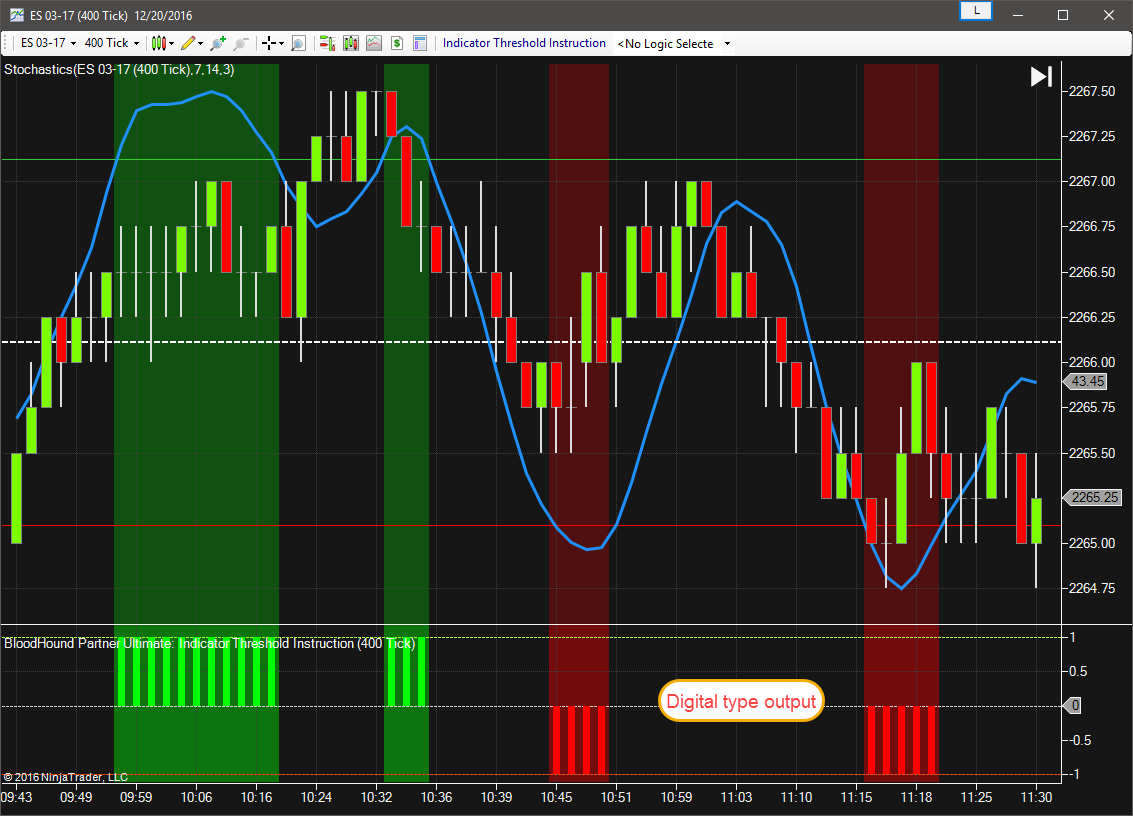#### Example 1C: The Basics of a Smooth Transition

This demonstrates the basic function of Indicator Threshold solver using the Stochastic D.  In this example the Solver’s output will transition between outputs always totaling 1.  As the Long output increases the Short output decreases, and vise versa.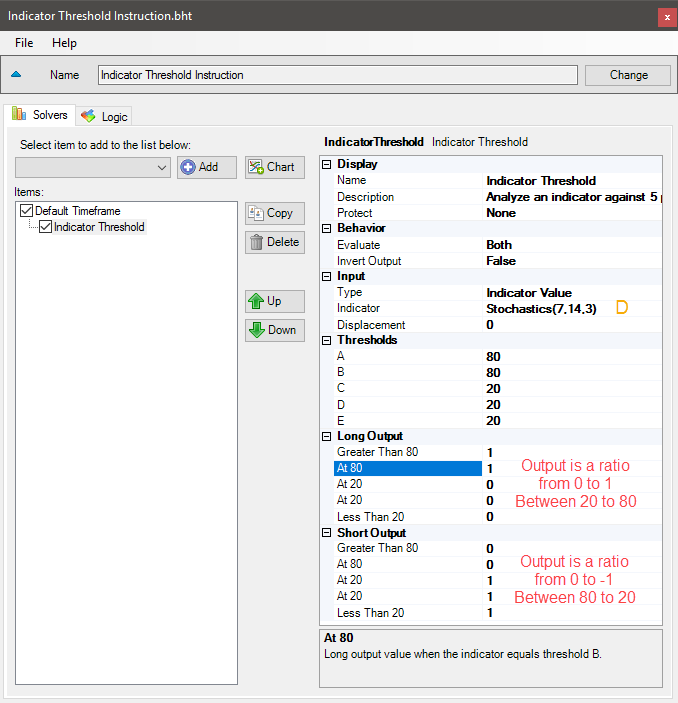• Add the Indicator Threshold solver
• Set Indicator to Stochastic D
• Set Thresholds values as shown
• Set Long Output > Greater Than 80 & At 80 to 1
• Set Short Output > At 20 & Less Than 20 to 1

The chart below shows a Long output when the Stochastic D is above 20, and a Short output when it is below 80.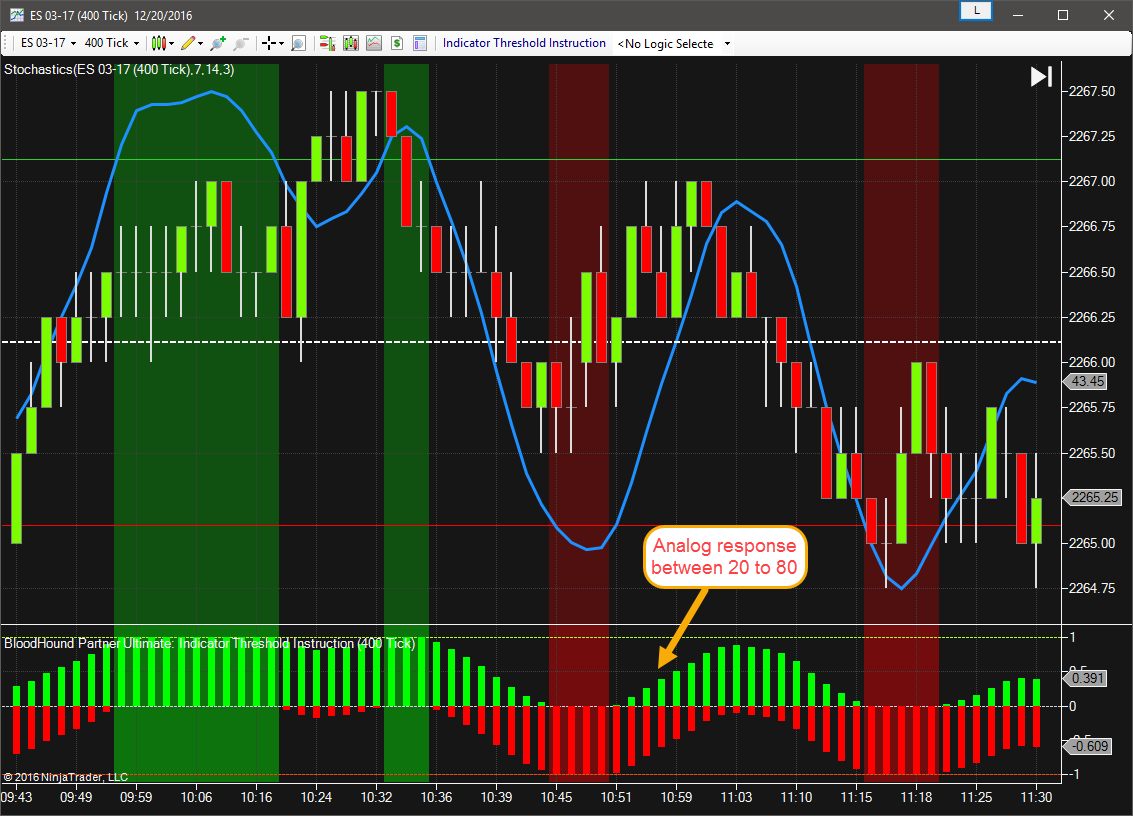#### Example 2: A Basic MACD Filter

This demonstrates the basic function of Indicator Threshold solver using the MACD line.  This Solver will be used to detect if the MACD is above the zero line for a Long(buy) filter, or below the zero line for a Short(sell) filter.  The zero line threshold of the MACD will be used to switch the output between Long and Short.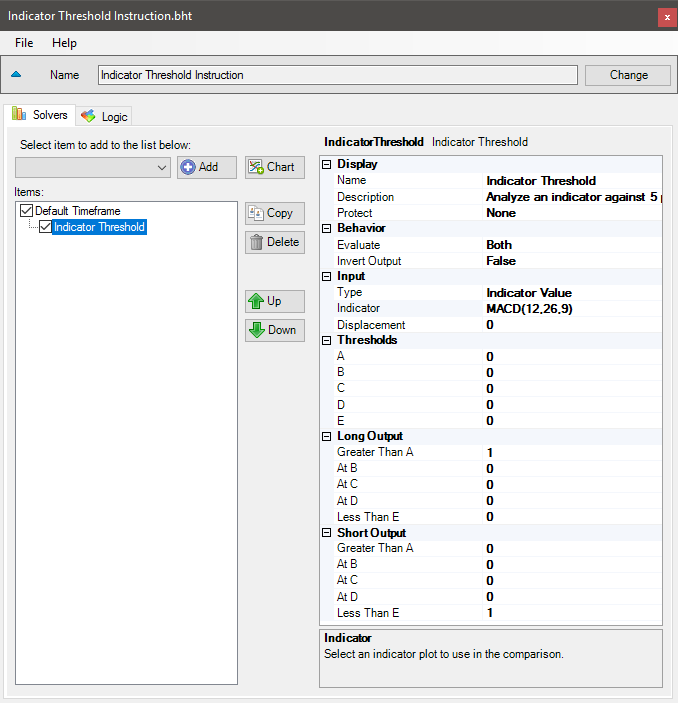• Add the Indicator Threshold solver
• Set Indicator to the MACD
• Leave the Thresholds values at zero
• Set Long Output > Greater Than A to 1
• Set Short Output > Less Than E to 1

The chart below shows a Long output when the MACD is above zero, and a Short output when it is below zero.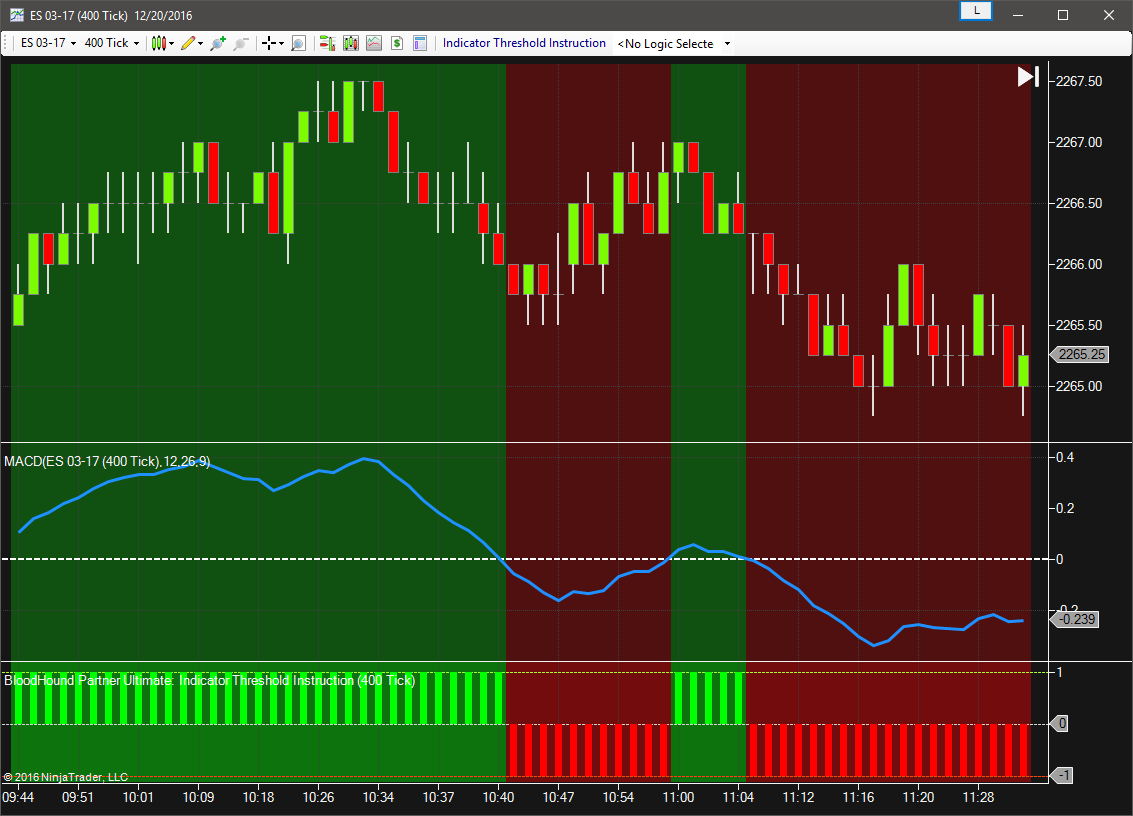#### Risk Disclosure

Futures, foreign currency and options trading contains substantial risk and is not for every investor. An investor could potentially lose all or more than the initial investment. Risk capital is money that can be lost without jeopardizing ones financial security or lifestyle. Only risk capital should be used for trading and only those with sufficient risk capital should consider trading. Past performance is not necessarily indicative of future results. View full risk disclosure.

## Indicator Threshold SolverThe Threshold solver compares an indicator against 4 possible threshold values. When the indicator value falls between thresholds a favorable value is generated calculated by linearly interpolated between the corresponding Output values.

### Parameters

#### Thresholds

Please note, Threshold values must be entered in descending order.
I.E  #1  A = 5, B = 4, C = 3, D = 2, E = 1
#2  A = 2, B = 1, C = 0, D =  -1, E =  -2

#### Short Output

Sets the solver’s Short output values as described in Long Output above.

### Video Tutorial

This video is from our weekly Workshop on July 10th, 2015.
For more benefit please watch in full screen mode, as this video is recorded in HD.

### Examples

#### Example 1A: The Basics

This demonstrates the basic function of Indicator Threshold solver using the Stochastic D.    This Solver will be used to detect over-bought, above 80, and over-sold, below 20, conditions of the Stochastic D.    In this first example the 50 line of the Stochastic will be used to switch the output between Long and Short.• Add the Indicator Threshold solver
• Set Indicator to Stochastic D
• Set Thresholds values as shown
• Set Long Output > Greater Than 80 to 1
• Set Short Output > Less Than 20 to 1

The chart below shows a Long output when the Stochastic D is above the 50 line, and a Short output when it is below the 50 line.#### Example 1B: The Basics of Going Digital

This demonstrates the basic function of Indicator Threshold solver using the Stochastic D.    This time the Solver will be set to give a digital style output.• Add the Indicator Threshold solver
• Set Indicator to Stochastic D
• Set Thresholds values as shown
• Set Long Output > Greater Than 80 to 1
• Set Short Output > Less Than 20 to 1

Notice that At B & At C are set to 0, and thus no output values between 20 to 80.    The chart below only shows values above 80 and below 20.#### Example 1C: The Basics of a Smooth Transition

This demonstrates the basic function of Indicator Threshold solver using the Stochastic D.  In this example the Solver’s output will transition between outputs always totaling 1.  As the Long output increases the Short output decreases, and vise versa.• Add the Indicator Threshold solver
• Set Indicator to Stochastic D
• Set Thresholds values as shown
• Set Long Output > Greater Than 80 & At 80 to 1
• Set Short Output > At 20 & Less Than 20 to 1

The chart below shows a Long output when the Stochastic D is above 20, and a Short output when it is below 80.#### Example 2: A Basic MACD Filter

This demonstrates the basic function of Indicator Threshold solver using the MACD line.  This Solver will be used to detect if the MACD is above the zero line for a Long(buy) filter, or below the zero line for a Short(sell) filter.  The zero line threshold of the MACD will be used to switch the output between Long and Short.• Add the Indicator Threshold solver
• Set Indicator to the MACD
• Leave the Thresholds values at zero
• Set Long Output > Greater Than A to 1
• Set Short Output > Less Than E to 1

The chart below shows a Long output when the MACD is above zero, and a Short output when it is below zero.